# Pagans

Jano and Michael ate pagans. Jano ate three more than Michael. The product of their counts (numbers) is 180. How many pagans did each of them eat?

Correct result:

j =  15
m =  12

#### Solution:

Our quadratic equation calculator calculates it.

$m=j-3=15-3=12$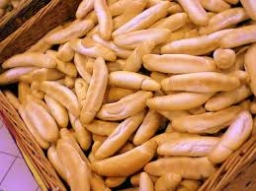We would be pleased if you find an error in the word problem, spelling mistakes, or inaccuracies and send it to us. Thank you!Tips to related online calculators

## Next similar math problems:

• Collection of stampsJano, Rado, and Fero have created a collection of stamps in a ratio of 5: 6: 9. Two of them had 429 stamps together. How many stamps did their shared collection have?
• Savings 2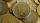Jozef and Michael saved 46 euros together. Michael saved 22 euros more than Jozef. How much did save each of them?
• Euler problem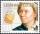Someone buys a 180 tolars towels. If it was for the same money of 3 more towels, it would be 3 tolars cheaper each. How many were towels?
• Find twoFind two consecutive natural numbers whose product is 1 larger than their sum. Searched numbers expressed by a fraction whose numerator is the difference between these numbers and the denominator is their sum.
• Chicken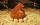I threw three chicken for a handful of grains and I noticed that it eat in a ratio of 8:7:6 and two of them grabbed 156 grains. How many grains have fought that chicken?
• Sweets, candyGrandfather gave out sweets to four children. At the last moment, two more children came, so in order to have them all the same, each of the four children would receive four candies less than they would have received if they had not. How much did my grand
• Lookout towerHow high is the lookout tower? If each step was 3 cm lower, there would be 60 more of them on the lookout tower. If it was 3 cm higher again, it would be 40 less than it is now.
• Fruit candies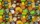Zuzka and Janka ate fruit candies. Zuzka ate 10 and Janka ate 3 times more. How many sweets did both girls eat together?
• Three brothersThe three brothers have a total of 42 years. Jan is five years younger than Peter and Peter is 2 years younger than Michael. How many years has each of them?
• Part-time workersThree part-time workers received CZK 1,235 for their work. The first received 20% less than the second and the third received 45 CZK more than the second. Determine how many crowns (CZK) each of them received.
• Product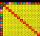The sum and the product of three integers is 6. Write largest of them.
• Trees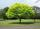Loggers wanted to seed more than 700 and less than 800 trees. If they seed in rows of 37, left them 8 trees. If they seed in rows of 43, left the 11 trees. How many trees must seed ?
• Pie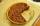Vili ate three pieces of pie . If it piece is 1/8 how much pie did he eat?
• Pine's forest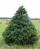There were so many pines in the forest that if they were sequentially numbered 1, 2, 3,. .. , would use three times more digits than the pine trees alone. How many pine trees were there in the forest?
• MO Z8-I-1 2018Fero and David meet daily in the elevator. One morning they found that if they multiply their current age, they get 238. If they did the same after four years, this product would be 378. Determine the sum of the current ages of Fero and David.
• Four integersFnd four consecutive integers so that the product of the first two is 70 times smaller than the product of the next two.
• Cows and calves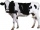There are 168 cows and calves in the cowshed. Cows are in nine stalls and calves in four stalls. Same count cows are in every cow stall and three more in each calf stall than in a cow stall. What is the capacity of the stalls for cows and what for calves?